# 从量化交易到资产管理—绝对收益之CTA策略开发

Author: Hukybo, Created: 2019-06-26 10:27:41, Updated: 2019-12-16 10:57:11

[TOC]

#### 1、期货CTA策略赚钱逻辑

##### 1.1 认识期货CTA

CTA基金管理规模变动情况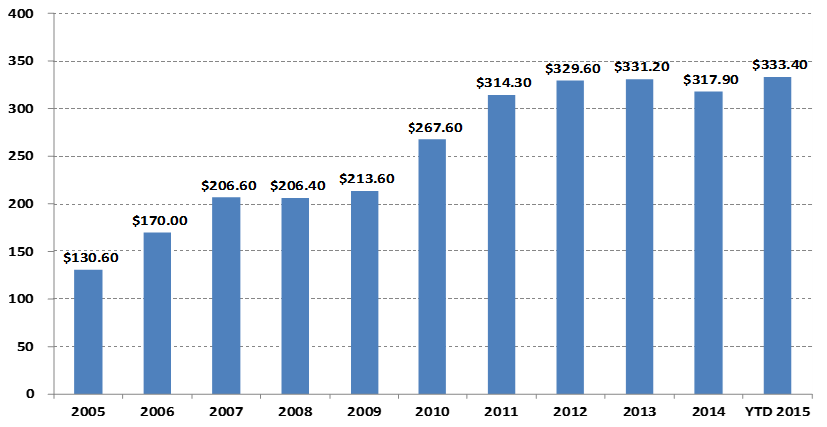单位：十亿美元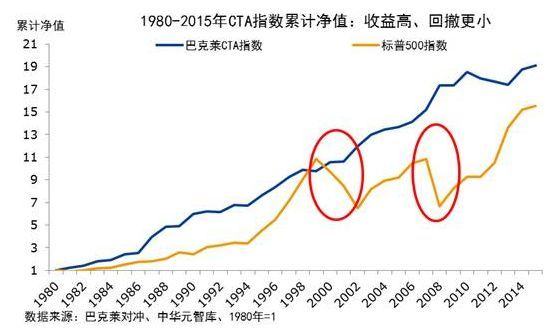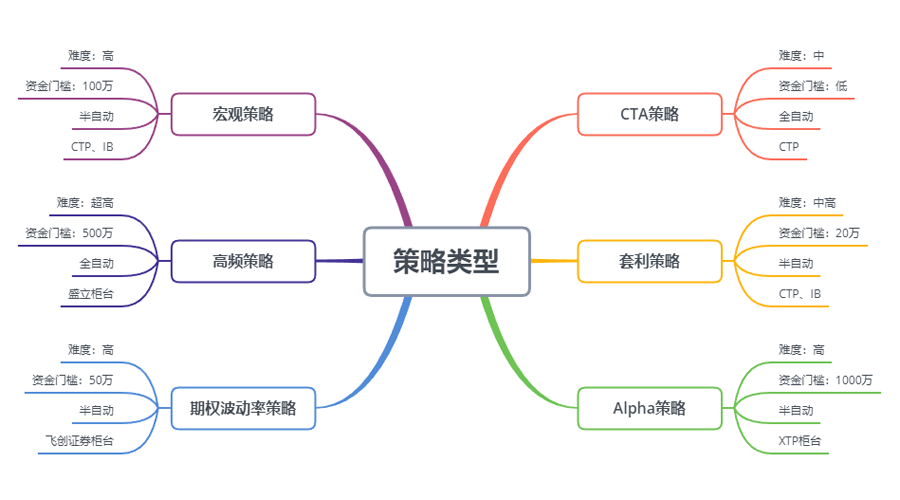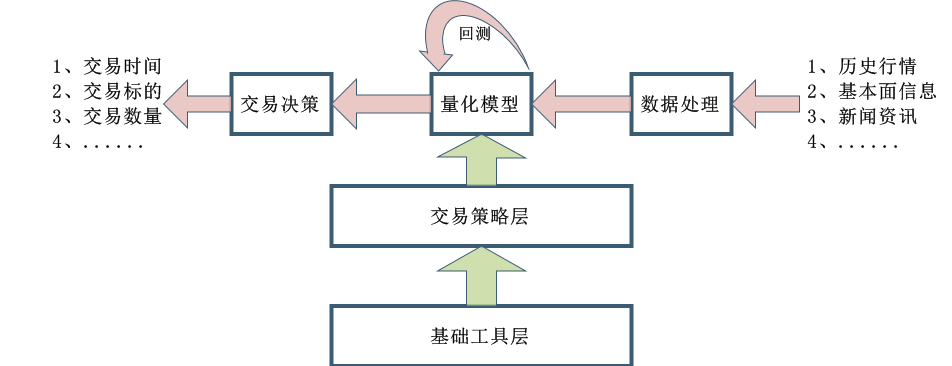CTA策略的设计流程也相对简单，首先把历史数据进行初步处理，然后输入到量化模型中，量化模型包括了数学建模、编程设计等工具形成的交易策略，通过计算分析这些数据产生交易信号。当然在实际开发中，并不像上图中那么简单，这里只是让大家有个整体的概念。

##### 1.2 期货CTA策略类型

CTA策略有不同的分类方法，根据交易方法，可以分为：主观交易和系统交易，国外的CTA策略发展的比较先进，系统交易的CTA策略已经接近100%。根据分析方法，可以分为：基本面分析和技术分析。根据收益来源，可以分为：趋势交易和震荡交易。

CTA策略数据 一般来说CTA策略是以分钟、小时以及日线数据为研究对象，其中数据包括：开盘价、最高价、最低价、收盘价、成交量等等；只有少部分CTA策略会用到Tick数据，比如L2数据中的买价、卖价、买量、卖量等深度数据。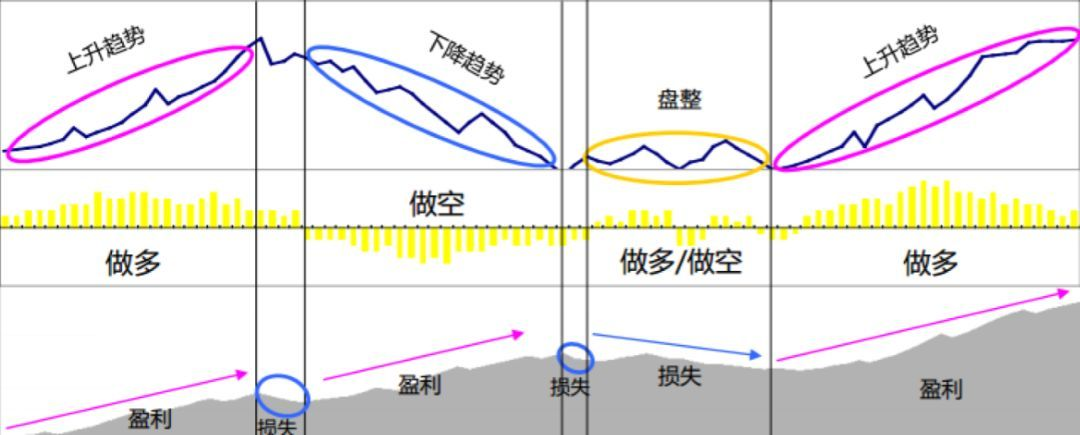CTA策略最大的优点是，不管当前市场是上涨还是下跌都能获得绝对收益，特别是在市场牛熊快速转换，或者行情走势明显流畅时，这种策略的优势是非常大的，总之就是有趋势在收益就有。但是如果市场处于震荡行情，或者趋势不明显时，这种策略就会可能买在高点卖在低点，不停的来回止损。

##### 1.3 期货CTA策略盈利原理

1. 价格走势存在反身性，它总是以趋势的方式不断延续。当投资者观察到价格上升的时候，就会跟风买入，结果造成价格进一步上升。价格下跌也是同样的道理。由于投资者更多的是非理性行为，所以有时候我们会看到，价格涨时涨得离谱，跌时跌的离谱。
2. 每一位投资者对盈利和亏损比例的容忍性是非对称的，对风险的承受能力也是不一样的。对于大多数散户来说，他们更倾向于选择更保守的顺势交易方法，市场也更容易走势趋势行情。
3. 价格的形成是由成交决定的，真是成交的背后又都是人来推动的，但人性是很难改变的，这就导致固定形态会反复出现的原因，策略在历史数据上回测有效，也就预示着将来可能也会有效。

CTA与传统资产的相关性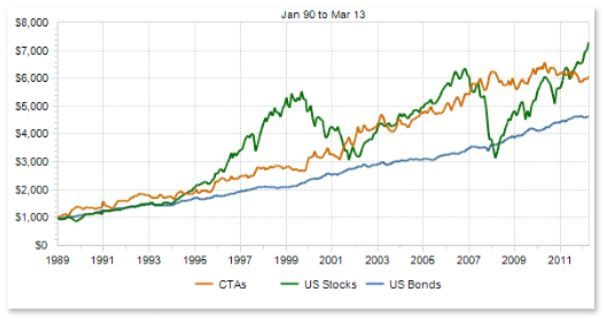#### 2、经典期货CTA策略案例

##### 2.1 期货基本面分析（库存、基差、价格）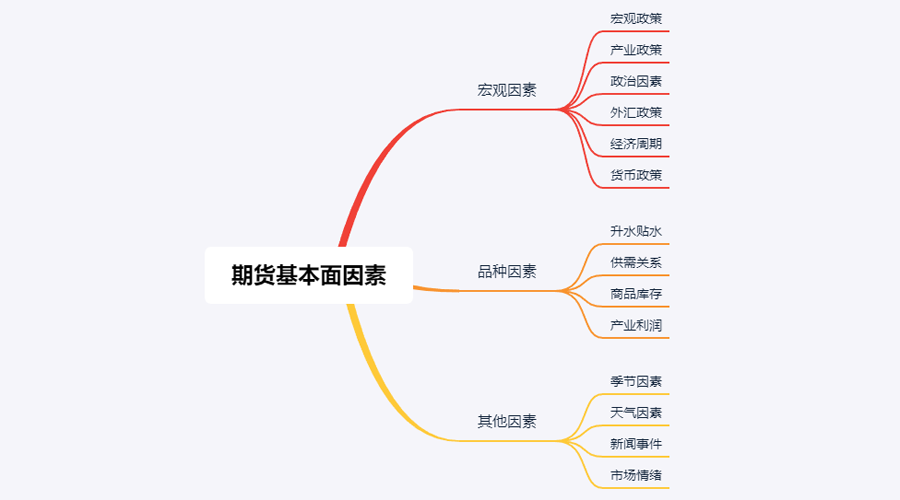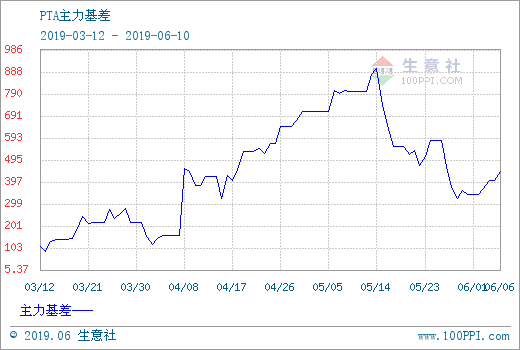##### 2.2 海龟交易法则

• 掌握优势：找到一个期望值为正的交易策略，因为从长期来看，它能创造正的回报。
• 管理风险：控制风险，守住阵地，否则你可能等不到创造成果的一天。
• 坚定不移：唯有坚定不移地执行你的策略，你才能真正获得系统的成效。
• 简单明了：从长久来看，简单的系统比复杂的系统更有生命力。

#### 3、实战开发期货CTA策略

##### 3.1 基于麦语言的CTA趋势策略开发

• 鳄鱼线(Alligator)

• 碎形(The Fractal)

• 动量(The Momentum)

• 加速(Acceleration)

• 均衡线(The Balance Line)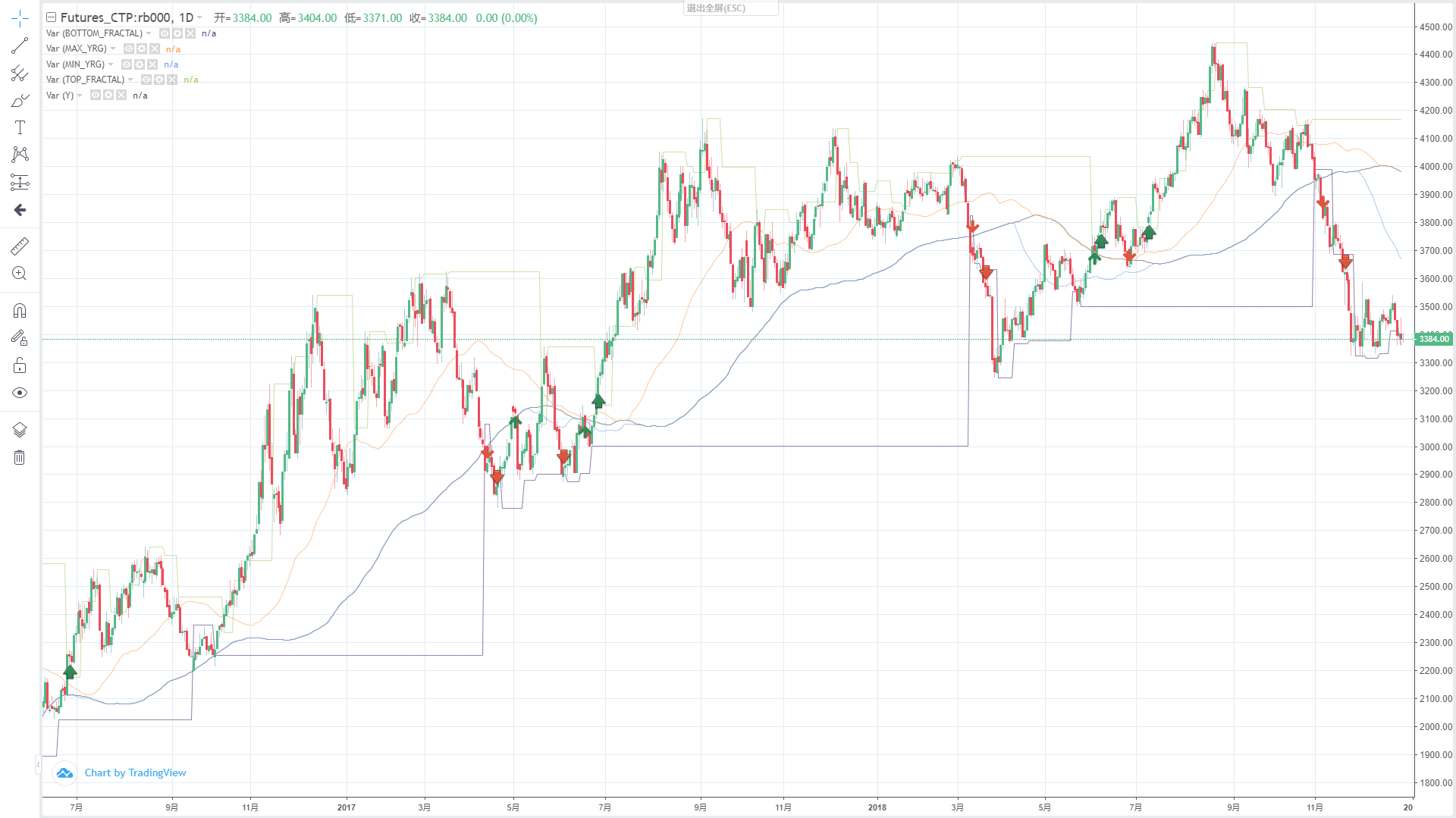``````// 参数
N1:=11;
N2:=21;

// 定义价格中线
N3:=N1+N2;
N4:=N2+N3;
HL:=(H+L)/2;

// 鳄鱼线
Y^^SMA(REF(HL,N3),N4,1);
R:=SMA(REF(HL,N2),N3,1);
G:=SMA(REF(HL,N1),N2,1);
``````

``````// 分形
TOP_N:=BARSLAST(REF(H,2)=HHV(H,5))+2;
BOTTOM_N:=BARSLAST(REF(L,2)=LLV(L,5))+2;

TOP:=REF(H,TOP_N);
BOTTOM:=REF(L,BOTTOM_N);

MAX_YRG^^MAX(MAX(Y,R),G);
MIN_YRG^^MIN(MIN(Y,R),G);

TOP_FRACTAL^^VALUEWHEN(H>=MAX_YRG,TOP);
BOTTOM_FRACTAL^^VALUEWHEN(L<=MIN_YRG,BOTTOM);
``````

``````// 如果当前无多单，并且收盘价升破上分形，并且上分形在鳄鱼线上方时，多头开仓
BKVOL=0 AND C>=TOP_FRACTAL AND TOP_FRACTAL>MAX_YRG,BPK(1);
// 如果当前无空单，并且收盘价跌破下分形，并且下分形在鳄鱼线下方时，空头开仓
SKVOL=0 AND C<=BOTTOM_FRACTAL AND BOTTOM_FRACTAL<MIN_YRG,SPK(1);

// 如果收盘价跌破鳄鱼的下巴时，多头平仓
C<Y,SP(BKVOL);
// 如果收盘价升破鳄鱼的下巴时，空头平仓
C>Y,BP(SKVOL);
``````

• 多头开仓：如果当前无多单，并且收盘价升破上分形，并且上分形在鳄鱼线上方。
• 空头开仓：如果当前无空单，并且收盘价跌破下分形，并且下分形在鳄鱼线下方。
• 多头平仓：如果收盘价跌破鳄鱼下巴。
• 空头平仓：如果收盘价升破鳄鱼下巴。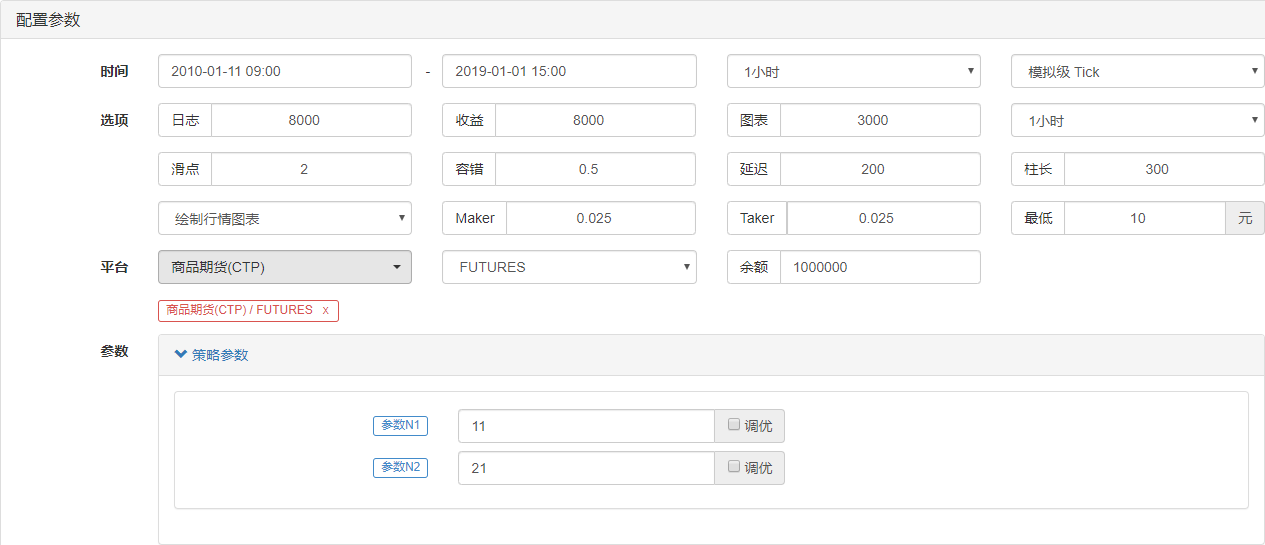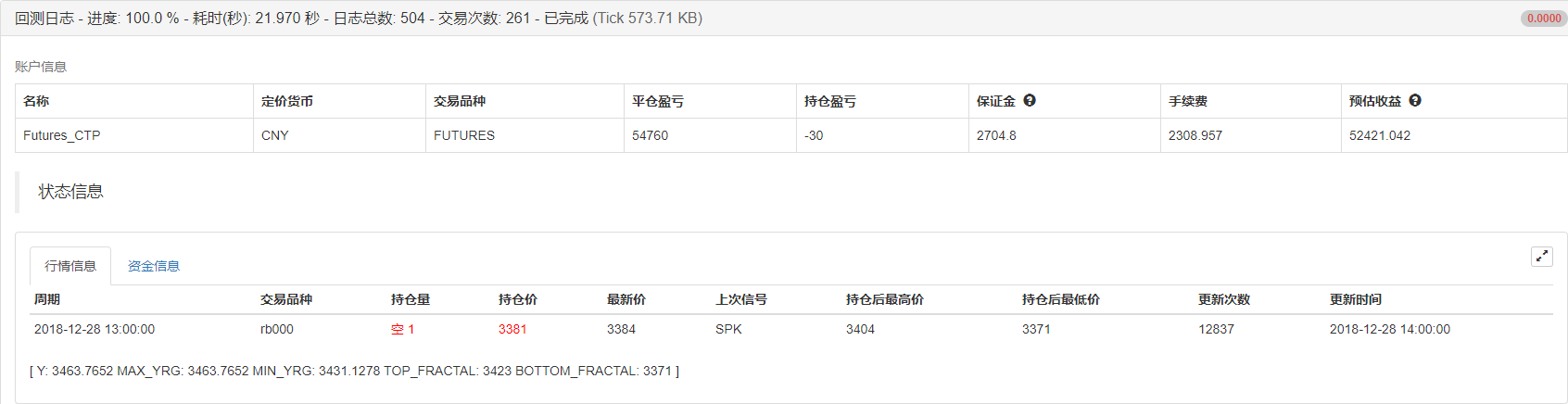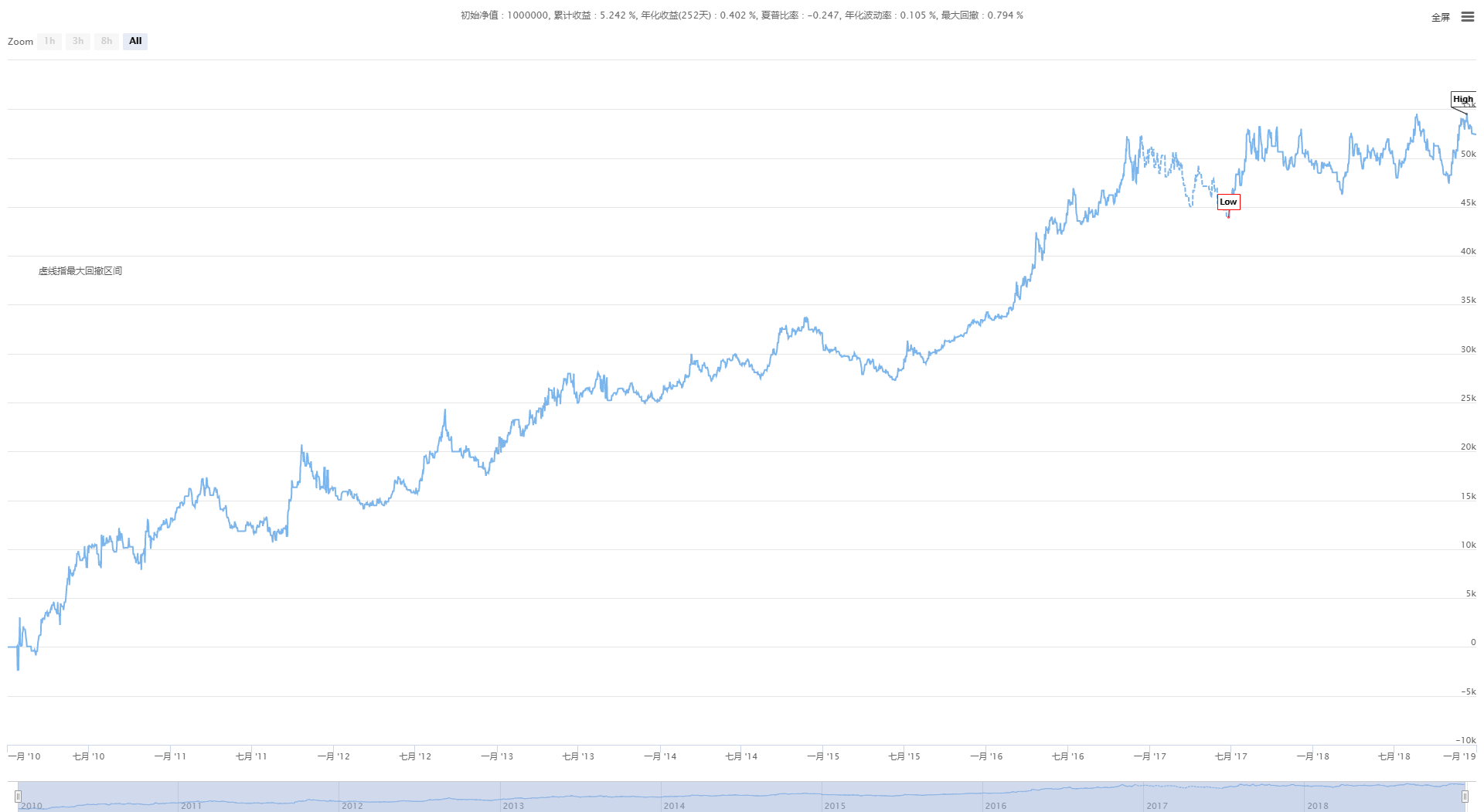##### 3.2 基于JavaScript语言的CTA套利策略开发

• 交易标的：以太经典（ETC）
• 价差数据：ETC 当周 - ETC 季度（省略协整性检验）
• 交易周期：5 分钟
• 头寸匹配：1:1
• 交易类型：同品种跨期

• 做多价差开仓条件：如果当前账户没有持仓，并且价差小于 boll 下轨，就做多价差。即：买开 ETC 当周，卖开 ETC 季度。
• 做空价差开仓条件：如果当前账户没有持仓，并且价差大于 boll 上轨，就做空价差。即：卖开 ETC 当周，买开 ETC 季度。
• 做多价差平仓条件：如果当前账户持有 ETC 当周多单，并且持有 ETC 季度空单，并且价差大于 boll 中轨，就平多价差。即：卖平 ETC 当周，买平 ETC 季度。
• 做空价差平仓条件：如果当前账户持有 ETC 当周空单，并且持有 ETC 季度多单，并且价差小于 boll 中轨，就平空价差。即：买平 ETC 当周，卖平 ETC 季度。

``````function Data() {}  // 基础数据函数
Data.prototype.mp = function () {}  // 持仓函数
Data.prototype.boll = function () {}  // 指标函数
Data.prototype.trade = function () {}  // 下单函数
Data.prototype.cancelOrders = function () {}  // 撤单函数
Data.prototype.isEven = function () {}  // 处理单只合约函数
Data.prototype.drawingChart = function () {}  // 画图函数

function onTick() {
var accountStocks = data.accountData.Stocks;  // 账户余额
var boll = data.boll(dataLength, timeCycle);  // 计算boll技术指标
data.cancelOrders();  // 撤单
data.drawingChart(boll);  // 画图
data.isEven();  // 处理持有单个合约
}

//入口函数
function main() {
while (true) {  // 进入轮询模式
onTick();  // 执行onTick函数
Sleep(500);  // 休眠0.5秒
}
}
``````

• 交易前预处理。
• 获取并计算数据。
• 下单并对后续处理。

1. 声明必要的全局变量

• 声明一个配置图表的 chart 对象 `var chart = {}`
• 调用 Chart 函数，初始化图表 `var ObjChart = Chart ( chart )`
• 声明一个空数组，用来存储价差序列 `var bars = []`
• 声明一个记录历史数据时间戳变量 `var oldTime = 0`

2. 配置策略的外部参数

``````var tradeTypeA = "this_week"; // 套利A合约
var tradeTypeB = "quarter"; // 套利B合约
var dataLength = 10; //指标周期长度
var timeCycle = 1; // K线周期
var name = "ETC"; // 币种
var unit = 1; // 下单量
``````

3. 定义数据处理函数

• 基础数据函数：Data ( ) 创建一个构造函数 Data，并定义它的内部属性。包括：账户数据、持仓数据、K线数据时间戳、套利A/B合约的买/卖一价、正/反套价差。
``````function Data(tradeTypeA, tradeTypeB) { // 传入套利A合约和套利B合约
this.accountData = _C(exchange.GetAccount); // 获取账户信息
this.positionData = _C(exchange.GetPosition); // 获取持仓信息
var recordsData = _C(exchange.GetRecords); //获取K线数据
var depthDataA = _C(exchange.GetDepth); // 套利A合约深度数据
var depthDataB = _C(exchange.GetDepth); // 套利B合约深度数据
this.time = recordsData[recordsData.length - 1].Time; // 获取最新数据时间
this.bidA = depthDataA.Bids.Price; // 套利A合约买一价
this.bidB = depthDataB.Bids.Price; // 套利B合约买一价
// 正套价差(合约A卖一价 - 合约B买一价)
// 反套价差(合约A买一价 - 合约B卖一价)
}
``````
• 获取持仓函数：mp ( ) 遍历整个持仓数组，返回指定合约、指定方向的持仓数量，如果没有就返回 false
``````Data.prototype.mp = function (tradeType, type) {
var positionData = this.positionData; // 获取持仓信息
for (var i = 0; i < positionData.length; i++) {
if (positionData[i].Type == type) {
if (positionData[i].Amount > 0) {
return positionData[i].Amount;
}
}
}
}
return false;
}
``````
• K线和指标函数：boll ( ) 根据正/反套价差数据，合成新的K线序列。并返回由boll指标计算的上轨、中轨、下轨数据。
``````Data.prototype.boll = function (num, timeCycle) {
var self = {}; // 临时对象
// 正套价差和反套价差中间值
self.Close = (this.basb + this.sabb) / 2;
if (this.timeA == this.timeB) {
self.Time = this.time;
} // 对比两个深度数据时间戳
if (this.time - oldTime > timeCycle * 60000) {
bars.push(self);
oldTime = this.time;
} // 根据指定时间周期，在K线数组里面传入价差数据对象
if (bars.length > num * 2) {
bars.shift(); // 控制K线数组长度
} else {
return;
}
var boll = TA.BOLL(bars, num, 2); // 调用talib库中的boll指标
return {
up: boll[boll.length - 1], // boll指标上轨
middle: boll[boll.length - 1], // boll指标中轨
down: boll[boll.length - 1] // boll指标下轨
} // 返回一个处理好的boll指标数据
}
``````
``````Data.prototype.trade = function (tradeType, type) {
bidPrice = this.bidA; // 设置bidPrice
bidPrice = this.bidB; // 设置bidPrice
}
switch (type) { // 匹配下单模式
exchange.SetDirection(type); // 设置下单模式
case "sell":
exchange.SetDirection(type); // 设置下单模式
return exchange.Sell(bidPrice, unit);
exchange.SetDirection(type); // 设置下单模式
return exchange.Sell(bidPrice, unit);
case "closesell":
exchange.SetDirection(type); // 设置下单模式
default:
return false;
}
}
``````
• 取消订单函数：cancelOrders ( ) 获取所有未成交订单数组，并逐个取消。并且如果有未成交的订单就返回false，如果没有未成交的订单就返回true。
``````Data.prototype.cancelOrders = function () {
Sleep(500); // 撤单前先延时，因为有些交易所你懂的
var orders = _C(exchange.GetOrders); // 获取未成交订单数组
if (orders.length > 0) { // 如果有未成交的订单
for (var i = 0; i < orders.length; i++) { //遍历未成交订单数组
exchange.CancelOrder(orders[i].Id); //逐个取消未成交的订单
Sleep(500); //延时0.5秒
}
return false; // 如果取消了未成交的单子就返回false
}
return true; //如果没有未成交的订单就返回true
}
``````
• 处理持有单个合约：isEven ( ) 在处理套利交易中出现单腿情况，这里直接用简单的平掉所有仓位处理。当然，也可以改为追单方式。
``````Data.prototype.isEven = function () {
var positionData = this.positionData; // 获取持仓信息
var type = null; // 转换持仓方向
// 如果持仓数组长度余2不等于0或者持仓数组长度不等于2
if (positionData.length % 2 != 0 || positionData.length != 2) {
for (var i = 0; i < positionData.length; i++) { // 遍历持仓数组
if (positionData[i].Type == 0) { // 如果是多单
type = 10; // 设置下单参数
} else if (positionData[i].Type == 1) { // 如果是空单
type = -10; // 设置下单参数
}
// 平掉所有仓位
}
}
}
``````
• 画图函数：drawingChart ( ) 调用 ObjChart.add ( ) 方法，在图表中画出必要的行情数据和指标数据：上轨、中轨、下轨、正/反套价差。
``````Data.prototype.drawingChart = function (boll) {
var nowTime = new Date().getTime();
ObjChart.update(chart);
}
``````

4. 在入口函数 main ( ) 里面，执行交易前预处理代码，这些代码在程序启动后，只运行一次。包括：

• 过滤控制台中不是很重要的信息 `SetErrorFilter ( )`
• 设置要交易的数字货币币种 `exchange.IO ( )`
• 程序启动前清空之前绘制的图表 `ObjChart.reset ( )`
• 程序启动前清空之前的状态栏信息 `LogProfitReset ( )`

``````function main() {
// 过滤控制台中不是很重要的信息
exchange.IO("currency", name + '_USDT'); //设置要交易的数字货币币种
ObjChart.reset(); //程序启动前清空之前绘制的图表
LogProfitReset(); //程序启动前清空之前的状态栏信息
while (true) { // 进入轮询模式
onTick(); // 执行onTick函数
Sleep(500); // 休眠0.5秒
}
}
``````

1. 获取基础数据对象、账户余额、boll 指标数据，以供交易逻辑使用。
``````function onTick() {
var accountStocks = data.accountData.Stocks; // 账户余额
var boll = data.boll(dataLength, timeCycle); // 获取boll指标数据
if (!boll) return; // 如果没有boll数据就返回
}
``````

1. 根据上述的策略逻辑，执行买卖操作。首先会判断价格和指标条件是否成立，然后再判断持仓条件是否成立，最后执行 trade ( ) 下单函数。
``````// 价差说明
// basb = (合约A卖一价 - 合约B买一价)
// sabb = (合约A买一价 - 合约B卖一价)
if (data.sabb > boll.middle && data.sabb < boll.up) { // 如果sabb高于中轨
if (data.mp(tradeTypeA, 0)) { // 下单前检测合约A是否有多单
}
if (data.mp(tradeTypeB, 1)) { // 下单前检测合约B是否有空单
}
} else if (data.basb < boll.middle && data.basb > boll.down) { // 如果basb低于中轨
if (data.mp(tradeTypeA, 1)) { // 下单前检测合约A是否有空单
}
if (data.mp(tradeTypeB, 0)) { // 下单前检测合约B是否有多单
}
}
if (data.basb < boll.down) { // 如果basb价差低于下轨
if (!data.mp(tradeTypeA, 0)) { // 下单前检测合约A是否有多单
}
if (!data.mp(tradeTypeB, 1)) { // 下单前检测合约B是否有空单
}
} else if (data.sabb > boll.up) { // 如果sabb价差高于上轨
if (!data.mp(tradeTypeA, 1)) { // 下单前检测合约A是否有空单
}
if (!data.mp(tradeTypeB, 0)) { // 下单前检测合约B是否有多单
}
}
}
``````
1. 下单完成后，需要对未成交的订单、持有单个合约等非正常情况做处理。以及绘制图表。
``````data.cancelOrders(); // 撤单
data.drawingChart(boll); // 画图
data.isEven(); // 处理持有单个合约
``````

``````// 全局变量
// 声明一个配置图表的 chart 对象
var chart = {
__isStock: true,
tooltip: {
xDateFormat: '%Y-%m-%d %H:%M:%S, %A'
},
title: {
text: '交易盈亏曲线图（详细）'
},
rangeSelector: {
buttons: [{
type: 'hour',
count: 1,
text: '1h'
}, {
type: 'hour',
count: 2,
text: '3h'
}, {
type: 'hour',
count: 8,
text: '8h'
}, {
type: 'all',
text: 'All'
}],
selected: 0,
inputEnabled: false
},
xAxis: {
type: 'datetime'
},
yAxis: {
title: {
text: '价差'
},
opposite: false,
},
series: [{
name: "上轨",
id: "线1,up",
data: []
}, {
name: "中轨",
id: "线2,middle",
data: []
}, {
name: "下轨",
id: "线3,down",
data: []
}, {
name: "basb",
id: "线4,basb",
data: []
}, {
name: "sabb",
id: "线5,sabb",
data: []
}]
};
var ObjChart = Chart(chart); // 画图对象
var bars = []; // 存储价差序列
var oldTime = 0; // 记录历史数据时间戳

// 参数
var tradeTypeA = "this_week"; // 套利A合约
var tradeTypeB = "quarter"; // 套利B合约
var dataLength = 10; //指标周期长度
var timeCycle = 1; // K线周期
var name = "ETC"; // 币种
var unit = 1; // 下单量

// 基础数据
this.accountData = _C(exchange.GetAccount); // 获取账户信息
this.positionData = _C(exchange.GetPosition); // 获取持仓信息
var recordsData = _C(exchange.GetRecords); //获取K线数据
var depthDataA = _C(exchange.GetDepth); // 套利A合约深度数据
var depthDataB = _C(exchange.GetDepth); // 套利B合约深度数据
this.time = recordsData[recordsData.length - 1].Time; // 获取最新数据时间
this.bidA = depthDataA.Bids.Price; // 套利A合约买一价
this.bidB = depthDataB.Bids.Price; // 套利B合约买一价
// 正套价差(合约A卖一价 - 合约B买一价)
// 反套价差(合约A买一价 - 合约B卖一价)
}

// 获取持仓
Data.prototype.mp = function (tradeType, type) {
var positionData = this.positionData; // 获取持仓信息
for (var i = 0; i < positionData.length; i++) {
if (positionData[i].Type == type) {
if (positionData[i].Amount > 0) {
return positionData[i].Amount;
}
}
}
}
return false;
}

// 合成新K线数据和boll指标数据
Data.prototype.boll = function (num, timeCycle) {
var self = {}; // 临时对象
// 正套价差和反套价差中间值
self.Close = (this.basb + this.sabb) / 2;
if (this.timeA == this.timeB) {
self.Time = this.time;
} // 对比两个深度数据时间戳
if (this.time - oldTime > timeCycle * 60000) {
bars.push(self);
oldTime = this.time;
} // 根据指定时间周期，在K线数组里面传入价差数据对象
if (bars.length > num * 2) {
bars.shift(); // 控制K线数组长度
} else {
return;
}
var boll = TA.BOLL(bars, num, 2); // 调用talib库中的boll指标
return {
up: boll[boll.length - 1], // boll指标上轨
middle: boll[boll.length - 1], // boll指标中轨
down: boll[boll.length - 1] // boll指标下轨
} // 返回一个处理好的boll指标数据
}

// 下单
bidPrice = this.bidA; // 设置bidPrice
bidPrice = this.bidB; // 设置bidPrice
}
switch (type) { // 匹配下单模式
exchange.SetDirection(type); // 设置下单模式
case "sell":
exchange.SetDirection(type); // 设置下单模式
return exchange.Sell(bidPrice, unit);
exchange.SetDirection(type); // 设置下单模式
return exchange.Sell(bidPrice, unit);
case "closesell":
exchange.SetDirection(type); // 设置下单模式
default:
return false;
}
}

// 取消订单
Data.prototype.cancelOrders = function () {
Sleep(500); // 撤单前先延时，因为有些交易所你懂的
var orders = _C(exchange.GetOrders); // 获取未成交订单数组
if (orders.length > 0) { // 如果有未成交的订单
for (var i = 0; i < orders.length; i++) { //遍历未成交订单数组
exchange.CancelOrder(orders[i].Id); //逐个取消未成交的订单
Sleep(500); //延时0.5秒
}
return false; // 如果取消了未成交的单子就返回false
}
return true; //如果没有未成交的订单就返回true
}

// 处理持有单个合约
Data.prototype.isEven = function () {
var positionData = this.positionData; // 获取持仓信息
var type = null; // 转换持仓方向
// 如果持仓数组长度余2不等于0或者持仓数组长度不等于2
if (positionData.length % 2 != 0 || positionData.length != 2) {
for (var i = 0; i < positionData.length; i++) { // 遍历持仓数组
if (positionData[i].Type ==``````

More

xunfeng91 学习

Hukybo 一起学习